# Test: Tangent & Normal (Competition Level) - 1

## 21 Questions MCQ Test Mathematics (Maths) Class 12 | Test: Tangent & Normal (Competition Level) - 1

Description
This mock test of Test: Tangent & Normal (Competition Level) - 1 for JEE helps you for every JEE entrance exam. This contains 21 Multiple Choice Questions for JEE Test: Tangent & Normal (Competition Level) - 1 (mcq) to study with solutions a complete question bank. The solved questions answers in this Test: Tangent & Normal (Competition Level) - 1 quiz give you a good mix of easy questions and tough questions. JEE students definitely take this Test: Tangent & Normal (Competition Level) - 1 exercise for a better result in the exam. You can find other Test: Tangent & Normal (Competition Level) - 1 extra questions, long questions & short questions for JEE on EduRev as well by searching above.
QUESTION: 1

### The area of the triangle formed by the positive x–axis and the normal and the tangent to the circle x2 + y2 = 4 at (1,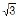) is

Solution:

x+ y= 4
Eqn of tangent at (1,√3)
xx1 + yy1 = 4
x + √3y = 4
√3y = −x + 4
y=−x / √3 + 4/√3
point A will be (4,0)
Eqn of Normal:slope of normal = √3
(y − √3) = √3 (x − 1)
=y − √3 = √3x − √3
y = √3x
Area of triangle = ∫(0 to 1)√3xdx + ∫(1 to 4)(−x/√3) + (4/√3)dx
=√3 [x/ 2] (0 to 1) + [(−x2) /(2√3) + 4x / √3] (1 to 4)
=√3.[1/2 − 0] + [−16 / 2√3 + 16 / √3 + 1/2 √3-4√3] = 8√3 − 7/2√3 + √3/2
=9 / 2√3 + 3 √2 = 12 / 2√3
= 2√3

QUESTION: 2

### Equation of the normal to the curve y = –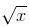+ 2 at the point of its intersection with the curve y = tan (tan–1 x) is

Solution:

y= −(x)1/2+2....(i)
And bisector of first quadrant is y=x ..... (ii)
On solving Eqs(i) and (ii) we get
x=1,4
∴ From Eq (i)
Points are (1,1) and (4,0)
But (4,0) not satisfy Eq (ii)
∴ Point (1,1) is only point of intersection of curve (i) and line (ii)
Now, slope of tangent of curve (i) is dy/dx = -1/(2(x)1/2)
∴ Slope of tangent at point (1,1) is −1/|dy/dx|(1,1) = -1/2
−1/|dy/dx|(1,1) = -1/(−1/2)
​= 2
∴ Equation of the normal to the curve at point (1,1) will be
y−1=2(x−1)
⇒2x−y−1=0

QUESTION: 3

### The abscissa of the point on the curve ay2 = x3, the normal at which cuts off equal intercepts from the coordinate axes is

Solution:

Slope of such normal is 1.⇒ dy/dx=1
ay= x3
⇒2ay dy/dx = 3x2
⇒ y=(3x2)/2a
⇒ a(3x2/2a)2
=x3
⇒x = 4a/9

QUESTION: 4

How many external tangents are there for two circles?

Solution:

External tangents are those which touch both the circles but they will not intersect in between the circles. The tangents touch at outmost points of circles that are ends of diameter if the circles have same diameter.

QUESTION: 5

If curve y = 1 – ax2 and y = x2 intersect orthogonally then the value of a is

Solution:
QUESTION: 6

The coordinates of the point of the parabola

y2 = 8x, which is at minimum distance from the circle x2+(y+6)2=1 are

Solution:
QUESTION: 7

The length of the subtangent to the curve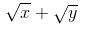=3 at the point (4, 1) is

Solution:

Length of subtangent =y(dx/dy)
Now given √x+√y=3
√y=3−√x
⇒1/2√ydy/dx = −1/(2√x) (On differentiating)
⇒dx/dy=−√x/√y
⇒ydx/dy=−√x/√y
⇒dx/dy/(4,1) = −√1√4
=−2
But the length can never be negative.
So length = 2.

QUESTION: 8

For a curve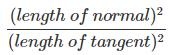is equal to

Solution:
QUESTION: 9

Water is poured into an inverted conical vessel of which the radius of the base is 2m and height 4m, at the rate of 77 litre/minute. The rate at which the water level is rising at the instant when the depth is 70 cm is: (use p = 22/7)

Solution:
QUESTION: 10

If the tangent at each point of the curve

y =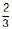x3 – 2ax2 + 2x + 5 makes an acute angle with the positive direction of x–axis, then

Solution:
QUESTION: 11

All points on the curve y2=4a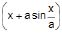at which the tangents are parallel to the axis of x, lie on a

Solution:

y2=4a[x+asin(x/a)] ..... (i)
∴2y dy/dx​=4a[1+cos(x/a)] ..... (ii)
If tangent is parallel to x-axis, then dy/dx=0
So, from Eq. (i), we get
cos(x/a)=−1
∴sin(x/a)=0
On putting this value in Eq. (i), we get
y2=4a(x+0)
⇒y2=4ax

QUESTION: 12

A curve is represented by the equations, x = sec2 t and y = cot t where t is a parameter. If the tangent at the point P on the curve where t = p/4 meets the curve again at the point Q then |PQ| is equal to

Solution:
QUESTION: 13

The curves x3 + p xy2 = –2 and 3 x2y – y3 = 2 are orthogonal for

Solution:
QUESTION: 14

If curves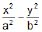= 1 and xy = c2 intersect orthogonally, then

Solution:

2x/a2+2y/b2−dy/dx=0
m1=dy/dx=−b2x/a2y
2x/A2−2y/B2⋅dy/dx=0
m2=dy/dx=B2x/A2y
m1⋅m2=−1
b2/a2⋅x/y⋅B2/A2⋅x/y=1
x2/y2=a2A2/b2B2
x^2/a2+y2/b2−x2/A2+y2/B2=0
x2(1/a2−1/A2)=−y2(1/b2+1/B2)
x2/y2=−(1/b2+1B2)/(1/a2−1/A2)
a2A2/b2B2=−(1/b2+1/B2)(1/a2−1/A2)
a2A2(1/a2−1/A2)=−b2B2(1/b2+1/B2)
a2A2(A2−a2/(a2B2))=−b2B2(B2+b2/(b2B2)
a2−A2=b2+B2
a2−b2=A2+B2

QUESTION: 15

The ordinate of y=(a/2) (ex/a + e–x/a) is the geometric mean of the length of the normal and the quantity

Solution:
QUESTION: 16

Angle between the tangents to the curve

y = x2 – 5x + 6 at the points (2, 0) and (3, 0) is

Solution:
QUESTION: 17

Water is being poured on to a cylindrical vessel at the rate of 1 m3/min. If the vessel has a circular base of radius 3m, the rate at which the level of water is rising in the vessel is

Solution:
QUESTION: 18

Find the number of points on the curve

x2 + y2 – 2x – 3 = 0 at which the tangents are parallel to the x-axis.

Solution:
QUESTION: 19

If at any point on a curve the subtangent and subnormal are equal, then the tangent is equal to

Solution:
QUESTION: 20

The number of values of c such that the straight line 3x + 4y = c touches the curve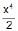= x + y is

Solution:
QUESTION: 21

The points(s) of intersection of the tangents drawn to the curve x2y = 1 – y at the points where it is intersected by the curve xy = 1 – y is/are given by

Solution: